Documentation

### This is machine translation

Mouseover text to see original. Click the button below to return to the English version of the page.

To view all translated materials including this page, select Country from the country navigator on the bottom of this page.

# pixelLabelImageDatastore

Datastore for semantic segmentation networks

## Description

Use `pixelLabelImageDatastore` to create a datastore for training a semantic segmentation network using deep learning.

## Creation

### Syntax

``pximds = pixelLabelImageDatastore(gTruth)``
``pximds = pixelLabelImageDatastore(imds,pxds)``
``pximds = pixelLabelImageDatastore(___,Name,Value)``

### Description

example

````pximds = pixelLabelImageDatastore(gTruth)` returns a datastore for training a semantic segmentation network based on the input `groundTruth` object or array of `groundTruth` objects. Use the output `pixelLabelImageDatastore` object with the Deep Learning Toolbox™ function `trainNetwork` to train convolutional neural networks for semantic segmentation.```
````pximds = pixelLabelImageDatastore(imds,pxds)` returns a datastore based on the input image datastore and the pixel label datastore objects. `imds` is an `ImageDatastore` object that represents the training input to the network. `pxds` is a `PixelLabelDatastore` object that represents the required network output.```
````pximds = pixelLabelImageDatastore(___,Name,Value)` additionally uses name-value pairs to set the `DispatchInBackground` and `OutputSizeMode` properties. For 2-D data, you can also use name-value pairs to specify the `ColorPreprocessing`, `DataAugmentation`, and `OutputSize` augmentation properties. You can specify multiple name-value pairs. Enclose each property name in quotes.For example, `pixelLabelImageDatastore(gTruth,'PatchesPerImage',40)` creates a pixel label image datastore that randomly generates 40 patches from each ground truth object in `gTruth`.```

### Input Arguments

expand all

Ground truth data, specified as a `groundTruth` object or as an array of `groundTruth` objects. Each `groundTruth` object contains information about the data source, the list of label definitions, and all marked labels for a set of ground truth labels.

Collection of images, specified as an `ImageDatastore` object.

Collection of pixel labeled images, specified as a `PixelLabelDatastore` object. The object contains the pixel labeled images for each image contained in the `imds` input object.

## Properties

expand all

This property is read-only.

Image file names used as the source for ground truth images, specified as a character vector or a cell array of character vectors.

This property is read-only.

Pixel label data file names used as the source for ground truth label images, specified as a character or a cell array of characters.

This property is read-only.

Class names, specified as a cell array of character vectors.

Color channel preprocessing for 2-D data, specified as `'none'`, `'gray2rgb'`, or `'rgb2gray'`. Use this property when you need the image data created by the data source must be only color or grayscale, but the training set includes both. Suppose you need to train a network that expects color images but some of your training images are grayscale. Set `ColorPreprocessing` to `'gray2rgb'` to replicate the color channels of the grayscale images in the input image set. Using the `'gray2rgb'` option creates M-by-N-by-3 output images.

The `ColorPreprocessing` property is not supported for 3-D data. To perform color channel preprocessing of 3-D data, use the `transform` function.

Preprocessing applied to input images, specified as an `imageDataAugmenter` object or `'none'`. When `DataAugmentation` is `'none'`, no preprocessing is applied to input images. Training data can be augmented in real-time during training.

The `DataAugmentation` property is not supported for 3-D data. To preprocess 3-D data, use the `transform` function.

Dispatch observations in the background during training, prediction, and classification, specified as `false` or `true`. To use background dispatching, you must have Parallel Computing Toolbox™. If `DispatchInBackground` is `true` and you have Parallel Computing Toolbox, then `pixelLabelImageDatastore` asynchronously reads patches, adds noise, and queues patch pairs.

This property is read-only.

Number of observations that are returned in each batch. For training, prediction, or classification, the `MiniBatchSize` property is set to the mini batch size defined in `trainingOptions`.

This property is read-only.

Total number of observations in the denoising image datastore. The number of observations is the length of one training epoch.

This property is read-only.

Size of output images, specified as a vector of two positive integers. The first element specifies the number of rows in the output images, and the second element specifies the number of columns. When you specify `OutputSize`, image sizes are adjusted as necessary. By default, this property is empty, which means that the images are not adjusted.

The `OutputSize` property is not supported for 3-D data. To set the output size of 3-D data, use the `transform` function.

Method used to resize output images, specified as one of the following. This property applies only when you set `OutputSize` to a value other than `[]`.

• `'resize'` — Scale the image to fit the output size. For more information, see `imresize`.

• `'centercrop'` — Take a crop from the center of the training image. The crop has the same size as the output size.

• `'randcrop'` — Take a random crop from the training image. The random crop has the same size as the output size.

Data Types: `char` | `string`

## Object Functions

 `combine` Combine data from multiple datastores `countEachLabel` Count occurrence of pixel label for data source images `hasdata` Determine if data is available to read `partitionByIndex` Partition pixelLabelImageDatastore according to indices `preview` Subset of data in datastore `read` Read data from pixelLabelImageDatastore `readall` Read all data in datastore `readByIndex` Read data specified by index from pixelLabelImageDatastore `reset` Reset datastore to initial state `shuffle` Shuffle data in pixelLabelImageDatastore `transform` Transform datastore

## Examples

collapse all

Load the training data.

```dataSetDir = fullfile(toolboxdir('vision'),'visiondata','triangleImages'); imageDir = fullfile(dataSetDir,'trainingImages'); labelDir = fullfile(dataSetDir,'trainingLabels');```

Create an image datastore for the images.

`imds = imageDatastore(imageDir);`

Create a `pixelLabelDatastore` for the ground truth pixel labels.

```classNames = ["triangle","background"]; labelIDs = [255 0]; pxds = pixelLabelDatastore(labelDir,classNames,labelIDs);```

Visualize training images and ground truth pixel labels.

```I = read(imds); C = read(pxds); I = imresize(I,5); L = imresize(uint8(C),5); imshowpair(I,L,'montage')```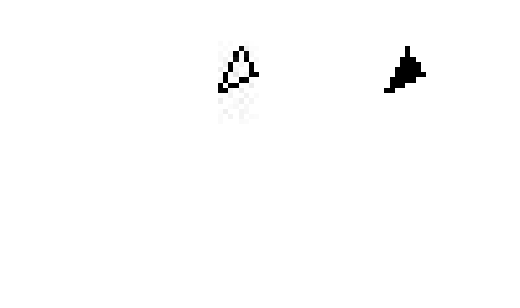Create a semantic segmentation network. This network uses a simple semantic segmentation network based on a downsampling and upsampling design.

```numFilters = 64; filterSize = 3; numClasses = 2; layers = [ imageInputLayer([32 32 1]) convolution2dLayer(filterSize,numFilters,'Padding',1) reluLayer() maxPooling2dLayer(2,'Stride',2) convolution2dLayer(filterSize,numFilters,'Padding',1) reluLayer() transposedConv2dLayer(4,numFilters,'Stride',2,'Cropping',1); convolution2dLayer(1,numClasses); softmaxLayer() pixelClassificationLayer() ]```
```layers = 10x1 Layer array with layers: 1 '' Image Input 32x32x1 images with 'zerocenter' normalization 2 '' Convolution 64 3x3 convolutions with stride [1 1] and padding [1 1 1 1] 3 '' ReLU ReLU 4 '' Max Pooling 2x2 max pooling with stride [2 2] and padding [0 0 0 0] 5 '' Convolution 64 3x3 convolutions with stride [1 1] and padding [1 1 1 1] 6 '' ReLU ReLU 7 '' Transposed Convolution 64 4x4 transposed convolutions with stride [2 2] and output cropping [1 1] 8 '' Convolution 2 1x1 convolutions with stride [1 1] and padding [0 0 0 0] 9 '' Softmax softmax 10 '' Pixel Classification Layer Cross-entropy loss ```

Setup training options.

```opts = trainingOptions('sgdm', ... 'InitialLearnRate',1e-3, ... 'MaxEpochs',100, ... 'MiniBatchSize',64);```

Create a pixel label image datastore that contains training data.

`trainingData = pixelLabelImageDatastore(imds,pxds);`

Train the network.

`net = trainNetwork(trainingData,layers,opts);`
```Training on single GPU. Initializing image normalization. |========================================================================================| | Epoch | Iteration | Time Elapsed | Mini-batch | Mini-batch | Base Learning | | | | (hh:mm:ss) | Accuracy | Loss | Rate | |========================================================================================| | 1 | 1 | 00:00:00 | 31.86% | 0.6934 | 0.0010 | | 17 | 50 | 00:00:03 | 94.52% | 0.5564 | 0.0010 | | 34 | 100 | 00:00:07 | 95.25% | 0.4415 | 0.0010 | | 50 | 150 | 00:00:11 | 95.14% | 0.3722 | 0.0010 | | 67 | 200 | 00:00:14 | 94.52% | 0.3336 | 0.0010 | | 84 | 250 | 00:00:18 | 95.25% | 0.2931 | 0.0010 | | 100 | 300 | 00:00:21 | 95.14% | 0.2708 | 0.0010 | |========================================================================================| ```

Read and display a test image.

```testImage = imread('triangleTest.jpg'); imshow(testImage)```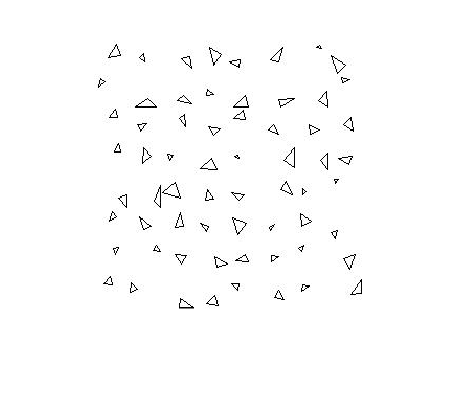Segment the test image and display the results.

```C = semanticseg(testImage,net); B = labeloverlay(testImage,C); imshow(B)```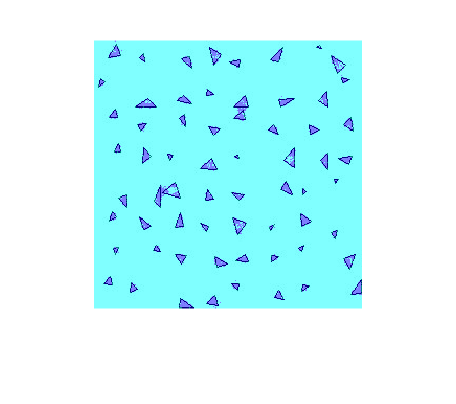Improve the results

The network failed to segment the triangles and classified every pixel as "background". The training appeared to be going well with training accuracies greater than 90%. However, the network only learned to classify the background class. To understand why this happened, you can count the occurrence of each pixel label across the dataset.

`tbl = countEachLabel(trainingData)`
```tbl=2×3 table Name PixelCount ImagePixelCount ____________ __________ _______________ 'triangle' 10326 2.048e+05 'background' 1.9447e+05 2.048e+05 ```

The majority of pixel labels are for the background. The poor results are due to the class imbalance. Class imbalance biases the learning process in favor of the dominant class. That's why every pixel is classified as "background". To fix this, use class weighting to balance the classes. There are several methods for computing class weights. One common method is inverse frequency weighting where the class weights are the inverse of the class frequencies. This increases weight given to under-represented classes.

```totalNumberOfPixels = sum(tbl.PixelCount); frequency = tbl.PixelCount / totalNumberOfPixels; classWeights = 1./frequency```
```classWeights = 2×1 19.8334 1.0531 ```

Class weights can be specified using the `pixelClassificationLayer`. Update the last layer to use a `pixelClassificationLayer` with inverse class weights.

`layers(end) = pixelClassificationLayer('Classes',tbl.Name,'ClassWeights',classWeights);`

Train network again.

`net = trainNetwork(trainingData,layers,opts);`
```Training on single GPU. Initializing image normalization. |========================================================================================| | Epoch | Iteration | Time Elapsed | Mini-batch | Mini-batch | Base Learning | | | | (hh:mm:ss) | Accuracy | Loss | Rate | |========================================================================================| | 1 | 1 | 00:00:00 | 47.50% | 0.6925 | 0.0010 | | 17 | 50 | 00:00:04 | 19.67% | 0.6837 | 0.0010 | | 34 | 100 | 00:00:08 | 75.77% | 0.4433 | 0.0010 | | 50 | 150 | 00:00:12 | 85.00% | 0.4018 | 0.0010 | | 67 | 200 | 00:00:16 | 87.00% | 0.3568 | 0.0010 | | 84 | 250 | 00:00:20 | 88.03% | 0.3153 | 0.0010 | | 100 | 300 | 00:00:24 | 90.42% | 0.2890 | 0.0010 | |========================================================================================| ```

Try to segment the test image again.

```C = semanticseg(testImage,net); B = labeloverlay(testImage,C); imshow(B)```Using class weighting to balance the classes produced a better segmentation result. Additional steps to improve the results include increasing the number of epochs used for training, adding more training data, or modifying the network.

Configure a pixel label image datastore to augment data while training.

Load training images and pixel labels.

```dataSetDir = fullfile(toolboxdir('vision'),'visiondata','triangleImages'); imageDir = fullfile(dataSetDir,'trainingImages'); labelDir = fullfile(dataSetDir,'trainingLabels');```

Create an `imageDatastore` object to hold the training images.

`imds = imageDatastore(imageDir);`

Define the class names and their associated label IDs.

```classNames = ["triangle","background"]; labelIDs = [255 0];```

Create a `pixelLabelDatastore` object to hold the ground truth pixel labels for the training images.

`pxds = pixelLabelDatastore(labelDir, classNames, labelIDs);`

Create an `imageDataAugmenter` object to randomly rotate and mirror image data.

`augmenter = imageDataAugmenter('RandRotation',[-10 10],'RandXReflection',true)`
```augmenter = imageDataAugmenter with properties: FillValue: 0 RandXReflection: 1 RandYReflection: 0 RandRotation: [-10 10] RandScale: [1 1] RandXScale: [1 1] RandYScale: [1 1] RandXShear: [0 0] RandYShear: [0 0] RandXTranslation: [0 0] RandYTranslation: [0 0] ```

Create a `pixelLabelImageDatastore` object to train the network with augmented data.

`plimds = pixelLabelImageDatastore(imds,pxds,'DataAugmentation',augmenter)`
```plimds = pixelLabelImageDatastore with properties: Images: {200x1 cell} PixelLabelData: {200x1 cell} ClassNames: {2x1 cell} DataAugmentation: [1x1 imageDataAugmenter] ColorPreprocessing: 'none' OutputSize: [] OutputSizeMode: 'resize' MiniBatchSize: 1 NumObservations: 200 DispatchInBackground: 0 ```

This example shows how to define and create a custom pixel classification layer that uses Dice loss.

This layer can be used to train semantic segmentation networks. To learn more about creating custom deep learning layers, see Define Custom Deep Learning Layers (Deep Learning Toolbox).

Dice Loss

The Dice loss is based on the Sørensen-Dice similarity coefficient for measuring overlap between two segmented images. The generalized Dice loss [1,2], $\mathit{L}$, for between one image $\mathit{Y}$ and the corresponding ground truth $\mathit{T}$ is given by

$\mathit{L}=1-\frac{2{\sum }_{\mathit{k}=1}^{\mathit{K}}{\mathit{w}}_{\mathit{k}}{\sum }_{\mathit{m}=1}^{\mathit{M}}{\mathit{Y}}_{\mathit{km}}{\mathit{T}}_{\mathit{km}}}{{\sum }_{\mathit{k}=1}^{\mathit{K}}{\mathit{w}}_{\mathit{k}}{\sum }_{\mathit{m}=1}^{\mathit{M}}{\mathit{Y}}_{\mathit{km}}^{2}+{\mathit{T}}_{\mathit{km}}^{2}}$ ,

where $\mathit{K}$ is the number of classes, $\mathit{M}$ is the number of elements along the first two dimensions of $\mathit{Y}$, and${\mathit{w}}_{\mathit{k}}$ is a class specific weighting factor that controls the contribution each class makes to the loss. ${\mathit{w}}_{\mathit{k}}$ is typically the inverse area of the expected region:

`${\mathit{w}}_{\mathit{k}}=\frac{1}{{\left(\sum _{\mathit{m}=1}^{\mathit{M}}{\mathit{T}}_{\mathit{km}}\right)}^{2}}$`

This weighting helps counter the influence of larger regions on the Dice score making it easier for the network to learn how to segment smaller regions.

Classification Layer Template

Copy the classification layer template into a new file in MATLAB®. This template outlines the structure of a classification layer and includes the functions that define the layer behavior. The rest of the example shows how to complete the `dicePixelClassificationLayer`.

```classdef dicePixelClassificationLayer < nnet.layer.ClassificationLayer properties % Optional properties end methods function loss = forwardLoss(layer, Y, T) % Layer forward loss function goes here. end function dLdY = backwardLoss(layer, Y, T) % Layer backward loss function goes here. end end end ```

Declare Layer Properties

By default, custom output layers have the following properties:

• `Name` – Layer name, specified as a character vector or a string scalar. To include this layer in a layer graph, you must specify a nonempty unique layer name. If you train a series network with this layer and `Name` is set to `''`, then the software automatically assigns a name at training time.

• `Description` – One-line description of the layer, specified as a character vector or a string scalar. This description appears when the layer is displayed in a `Layer` array. If you do not specify a layer description, then the software displays the layer class name.

• `Type` – Type of the layer, specified as a character vector or a string scalar. The value of `Type` appears when the layer is displayed in a `Layer` array. If you do not specify a layer type, then the software displays `'Classification layer'` or `'Regression layer'`.

Custom classification layers also have the following property:

• `Classes` – Classes of the output layer, specified as a categorical vector, string array, cell array of character vectors, or `'auto'`. If `Classes` is `'auto'`, then the software automatically sets the classes at training time. If you specify a string array or cell array of character vectors `str`, then the software sets the classes of the output layer to `categorical(str,str)`. The default value is `'auto'`.

If the layer has no other properties, then you can omit the `properties` section.

The Dice loss requires a small constant value to prevent division by zero. Specify the property, `Epsilon`, to hold this value.

```classdef dicePixelClassificationLayer < nnet.layer.ClassificationLayer properties(Constant) % Small constant to prevent division by zero. Epsilon = 1e-8; end ... end ```

Create Constructor Function

Create the function that constructs the layer and initializes the layer properties. Specify any variables required to create the layer as inputs to the constructor function.

Specify an optional input argument name to assign to the `Name` property at creation.

``` function layer = dicePixelClassificationLayer(name) % layer = dicePixelClassificationLayer(name) creates a Dice % pixel classification layer with the specified name. % Set layer name. layer.Name = name; % Set layer description. layer.Description = 'Dice loss'; end ```

Create Forward Loss Function

Create a function named `forwardLoss` that returns the weighted cross entropy loss between the predictions made by the network and the training targets. The syntax for `forwardLoss` is `loss = forwardLoss(layer, Y, T)`, where `Y` is the output of the previous layer and `T` represents the training targets.

For semantic segmentation problems, the dimensions of `T` match the dimension of `Y`, where `Y` is a 4-D array of size `H`-by-`W`-by-`K`-by-`N`, where `K` is the number of classes, and `N` is the mini-batch size.

The size of `Y` depends on the output of the previous layer. To ensure that `Y` is the same size as `T`, you must include a layer that outputs the correct size before the output layer. For example, to ensure that `Y` is a 4-D array of prediction scores for `K` classes, you can include a fully connected layer of size `K` or a convolutional layer with `K` filters followed by a softmax layer before the output layer.

``` function loss = forwardLoss(layer, Y, T) % loss = forwardLoss(layer, Y, T) returns the Dice loss between % the predictions Y and the training targets T. % Weights by inverse of region size. W = 1 ./ sum(sum(T,1),2).^2; intersection = sum(sum(Y.*T,1),2); union = sum(sum(Y.^2 + T.^2, 1),2); numer = 2*sum(W.*intersection,3) + layer.Epsilon; denom = sum(W.*union,3) + layer.Epsilon; % Compute Dice score. dice = numer./denom; % Return average Dice loss. N = size(Y,4); loss = sum((1-dice))/N; end ```

Create Backward Loss Function

Create the backward loss function that returns the derivatives of the Dice loss with respect to the predictions `Y`. The syntax for `backwardLoss` is `loss = backwardLoss(layer, Y, T)`, where `Y` is the output of the previous layer and `T` represents the training targets.

The dimensions of `Y` and `T` are the same as the inputs in `forwardLoss`.

``` function dLdY = backwardLoss(layer, Y, T) % dLdY = backwardLoss(layer, Y, T) returns the derivatives of % the Dice loss with respect to the predictions Y. % Weights by inverse of region size. W = 1 ./ sum(sum(T,1),2).^2; intersection = sum(sum(Y.*T,1),2); union = sum(sum(Y.^2 + T.^2, 1),2); numer = 2*sum(W.*intersection,3) + layer.Epsilon; denom = sum(W.*union,3) + layer.Epsilon; N = size(Y,4); dLdY = (2*W.*Y.*numer./denom.^2 - 2*W.*T./denom)./N; end ```

Completed Layer

The completed layer is provided in `dicePixelClassificationLayer.m`.

```classdef dicePixelClassificationLayer < nnet.layer.ClassificationLayer % This layer implements the generalized dice loss function for training % semantic segmentation networks. properties(Constant) % Small constant to prevent division by zero. Epsilon = 1e-8; end methods function layer = dicePixelClassificationLayer(name) % layer = dicePixelClassificationLayer(name) creates a Dice % pixel classification layer with the specified name. % Set layer name. layer.Name = name; % Set layer description. layer.Description = 'Dice loss'; end function loss = forwardLoss(layer, Y, T) % loss = forwardLoss(layer, Y, T) returns the Dice loss between % the predictions Y and the training targets T. % Weights by inverse of region size. W = 1 ./ sum(sum(T,1),2).^2; intersection = sum(sum(Y.*T,1),2); union = sum(sum(Y.^2 + T.^2, 1),2); numer = 2*sum(W.*intersection,3) + layer.Epsilon; denom = sum(W.*union,3) + layer.Epsilon; % Compute Dice score. dice = numer./denom; % Return average Dice loss. N = size(Y,4); loss = sum((1-dice))/N; end function dLdY = backwardLoss(layer, Y, T) % dLdY = backwardLoss(layer, Y, T) returns the derivatives of % the Dice loss with respect to the predictions Y. % Weights by inverse of region size. W = 1 ./ sum(sum(T,1),2).^2; intersection = sum(sum(Y.*T,1),2); union = sum(sum(Y.^2 + T.^2, 1),2); numer = 2*sum(W.*intersection,3) + layer.Epsilon; denom = sum(W.*union,3) + layer.Epsilon; N = size(Y,4); dLdY = (2*W.*Y.*numer./denom.^2 - 2*W.*T./denom)./N; end end end ```

GPU Compatibility

For GPU compatibility, the layer functions must support inputs and return outputs of type `gpuArray`. Any other functions used by the layer must do the same.

The MATLAB functions used in `forwardLoss`, and `backwardLoss` in `dicePixelClassificationLayer` all support `gpuArray` inputs, so the layer is GPU compatible.

Check Output Layer Validity

Create an instance of the layer.

`layer = dicePixelClassificationLayer('dice');`

Check the layer validity of the layer using `checkLayer`. Specify the valid input size to be the size of a single observation of typical input to the layer. The layer expects a `H`-by-`W`-by-`K`-by-`N` array inputs, where `K` is the number of classes, and `N` is the number of observations in the mini-batch.

```numClasses = 2; validInputSize = [4 4 numClasses]; checkLayer(layer,validInputSize, 'ObservationDimension',4)```
```Running nnet.checklayer.OutputLayerTestCase .......... ....... Done nnet.checklayer.OutputLayerTestCase __________ Test Summary: 17 Passed, 0 Failed, 0 Incomplete, 0 Skipped. Time elapsed: 1.6227 seconds. ```

The test summary reports the number of passed, failed, incomplete, and skipped tests.

Use Custom Layer in Semantic Segmentation Network

Create a semantic segmentation network that uses the `dicePixelClassificationLayer`.

```layers = [ imageInputLayer([32 32 1]) convolution2dLayer(3,64,'Padding',1) reluLayer maxPooling2dLayer(2,'Stride',2) convolution2dLayer(3,64,'Padding',1) reluLayer transposedConv2dLayer(4,64,'Stride',2,'Cropping',1) convolution2dLayer(1,2) softmaxLayer dicePixelClassificationLayer('dice')]```
```layers = 10x1 Layer array with layers: 1 '' Image Input 32x32x1 images with 'zerocenter' normalization 2 '' Convolution 64 3x3 convolutions with stride [1 1] and padding [1 1 1 1] 3 '' ReLU ReLU 4 '' Max Pooling 2x2 max pooling with stride [2 2] and padding [0 0 0 0] 5 '' Convolution 64 3x3 convolutions with stride [1 1] and padding [1 1 1 1] 6 '' ReLU ReLU 7 '' Transposed Convolution 64 4x4 transposed convolutions with stride [2 2] and output cropping [1 1] 8 '' Convolution 2 1x1 convolutions with stride [1 1] and padding [0 0 0 0] 9 '' Softmax softmax 10 'dice' Classification Output Dice loss ```

Load training data for semantic segmentation using `imageDatastore` and `pixelLabelDatastore`.

```dataSetDir = fullfile(toolboxdir('vision'),'visiondata','triangleImages'); imageDir = fullfile(dataSetDir,'trainingImages'); labelDir = fullfile(dataSetDir,'trainingLabels'); imds = imageDatastore(imageDir); classNames = ["triangle" "background"]; labelIDs = [255 0]; pxds = pixelLabelDatastore(labelDir, classNames, labelIDs);```

Associate the image and pixel label data using `pixelLabelImageDatastore`.

`ds = pixelLabelImageDatastore(imds,pxds);`

Set the training options and train the network.

```options = trainingOptions('sgdm', ... 'InitialLearnRate',1e-2, ... 'MaxEpochs',100, ... 'LearnRateDropFactor',1e-1, ... 'LearnRateDropPeriod',50, ... 'LearnRateSchedule','piecewise', ... 'MiniBatchSize',128); net = trainNetwork(ds,layers,options);```
```Training on single GPU. Initializing image normalization. |========================================================================================| | Epoch | Iteration | Time Elapsed | Mini-batch | Mini-batch | Base Learning | | | | (hh:mm:ss) | Accuracy | Loss | Rate | |========================================================================================| | 1 | 1 | 00:00:03 | 27.89% | 0.8346 | 0.0100 | | 50 | 50 | 00:00:34 | 89.67% | 0.6384 | 0.0100 | | 100 | 100 | 00:01:09 | 94.35% | 0.5024 | 0.0010 | |========================================================================================| ```

Evaluate the trained network by segmenting a test image and displaying the segmentation result.

```I = imread('triangleTest.jpg'); [C,scores] = semanticseg(I,net); B = labeloverlay(I,C); figure imshow(imtile({I,B}))```References

1. Crum, William R., Oscar Camara, and Derek LG Hill. "Generalized overlap measures for evaluation and validation in medical image analysis." IEEE transactions on medical imaging 25.11 (2006): 1451-1461.

2. Sudre, Carole H., et al. "Generalised Dice overlap as a deep learning loss function for highly unbalanced segmentations." Deep Learning in Medical Image Analysis and Multimodal Learning for Clinical Decision Support. Springer, Cham, 2017. 240-248.

This example shows how to train a semantic segmentation network using dilated convolutions.

A semantic segmentation network classifies every pixel in an image, resulting in an image that is segmented by class. Applications for semantic segmentation include road segmentation for autonomous driving and cancer cell segmentation for medical diagnosis. To learn more, see Semantic Segmentation Basics.

Semantic segmentation networks like DeepLab  make extensive use of dilated convolutions (also known as atrous convolutions) because they can increase the receptive field of the layer (the area of the input which the layers can see) without increasing the number of parameters or computations.

The example uses a simple dataset of 32x32 triangle images for illustration purposes. The dataset includes accompanying pixel label ground truth data. Load the training data using an `imageDatastore` and a `pixelLabelDatastore`.

```dataFolder = fullfile(toolboxdir('vision'),'visiondata','triangleImages'); imageFolderTrain = fullfile(dataFolder,'trainingImages'); labelFolderTrain = fullfile(dataFolder,'trainingLabels');```

Create an image datastore for the images.

`imdsTrain = imageDatastore(imageFolderTrain);`

Create a `pixelLabelDatastore` for the ground truth pixel labels.

```classNames = ["triangle" "background"]; labels = [255 0]; pxdsTrain = pixelLabelDatastore(labelFolderTrain,classNames,labels)```
```pxdsTrain = PixelLabelDatastore with properties: Files: {200×1 cell} ClassNames: {2×1 cell} ReadSize: 1 ReadFcn: @readDatastoreImage AlternateFileSystemRoots: {} ```

Create Semantic Segmentation Network

This example uses a simple semantic segmentation network based on dilated convolutions.

Create a data source for training data and get the pixel counts for each label.

```pximdsTrain = pixelLabelImageDatastore(imdsTrain,pxdsTrain); tbl = countEachLabel(pximdsTrain)```
```tbl=2×3 table Name PixelCount ImagePixelCount ____________ __________ _______________ 'triangle' 10326 2.048e+05 'background' 1.9447e+05 2.048e+05 ```

The majority of pixel labels are for background. This class imbalance biases the learning process in favor of the dominant class. To fix this, use class weighting to balance the classes. There are several methods for computing class weights. One common method is inverse frequency weighting where the class weights are the inverse of the class frequencies. This increases weight given to under-represented classes. Calculate the class weights using inverse frequency weighting.

```numberPixels = sum(tbl.PixelCount); frequency = tbl.PixelCount / numberPixels; classWeights = 1 ./ frequency;```

Create a network for pixel classificaiton with an image input layer with input size corresponding to the size of the input images. Next, specify three blocks of convolution, batch normalization, and ReLU layers. For each convolutional layer, specify 32 3-by-3 filters with increasing dilation factors and specify to pad the inputs to be the same size as the outputs by setting the `'Padding'` option to `'same'`. To classify the pixels, include a convolutional layer with K 1-by-1 convolutions, where K is the number of classes, followed by a softmax layer and a `pixelClassificationLayer` with the inverse class weights.

```inputSize = [32 32 1]; filterSize = 3; numFilters = 32; numClasses = numel(classNames); layers = [ imageInputLayer(inputSize) convolution2dLayer(filterSize,numFilters,'DilationFactor',1,'Padding','same') batchNormalizationLayer reluLayer convolution2dLayer(filterSize,numFilters,'DilationFactor',2,'Padding','same') batchNormalizationLayer reluLayer convolution2dLayer(filterSize,numFilters,'DilationFactor',4,'Padding','same') batchNormalizationLayer reluLayer convolution2dLayer(1,numClasses) softmaxLayer pixelClassificationLayer('Classes',classNames,'ClassWeights',classWeights)];```

Train Network

Specify the training options. Using the SGDM solver, train for 100 epochs, mini-batch size 64, and learn rate 0.001.

```options = trainingOptions('sgdm', ... 'MaxEpochs', 100, ... 'MiniBatchSize', 64, ... 'InitialLearnRate', 1e-3);```

Train the network using `trainNetwork`.

`net = trainNetwork(pximdsTrain,layers,options);`
```Training on single GPU. Initializing image normalization. |========================================================================================| | Epoch | Iteration | Time Elapsed | Mini-batch | Mini-batch | Base Learning | | | | (hh:mm:ss) | Accuracy | Loss | Rate | |========================================================================================| | 1 | 1 | 00:00:00 | 67.54% | 0.7098 | 0.0010 | | 17 | 50 | 00:00:03 | 84.60% | 0.3851 | 0.0010 | | 34 | 100 | 00:00:06 | 89.85% | 0.2536 | 0.0010 | | 50 | 150 | 00:00:09 | 93.39% | 0.1959 | 0.0010 | | 67 | 200 | 00:00:11 | 95.89% | 0.1559 | 0.0010 | | 84 | 250 | 00:00:14 | 97.29% | 0.1188 | 0.0010 | | 100 | 300 | 00:00:18 | 98.28% | 0.0970 | 0.0010 | |========================================================================================| ```

Test Network

Load the test data. Create an image datastore for the images. Create a `pixelLabelDatastore` for the ground truth pixel labels.

```imageFolderTest = fullfile(dataFolder,'testImages'); imdsTest = imageDatastore(imageFolderTest); labelFolderTest = fullfile(dataFolder,'testLabels'); pxdsTest = pixelLabelDatastore(labelFolderTest,classNames,labels);```

Make predictions using the test data and trained network.

`pxdsPred = semanticseg(imdsTest,net,'WriteLocation',tempdir);`
```Running semantic segmentation network ------------------------------------- * Processing 100 images. * Progress: 100.00% ```

Evaluate the prediction accuracy using `evaluateSemanticSegmentation`.

`metrics = evaluateSemanticSegmentation(pxdsPred,pxdsTest);`
```Evaluating semantic segmentation results ---------------------------------------- * Selected metrics: global accuracy, class accuracy, IoU, weighted IoU, BF score. * Processing 100 images... [==================================================] 100% Elapsed time: 00:00:00 Estimated time remaining: 00:00:00 * Finalizing... Done. * Data set metrics: GlobalAccuracy MeanAccuracy MeanIoU WeightedIoU MeanBFScore ______________ ____________ _______ ___________ ___________ 0.98334 0.99107 0.85869 0.97109 0.68197 ```

For more information on evaluating semantic segmentation networks, see `evaluateSemanticSegmentation`.

Segment New Image

Read and display the test image `triangleTest.jpg`.

```imgTest = imread('triangleTest.jpg'); figure imshow(imgTest)```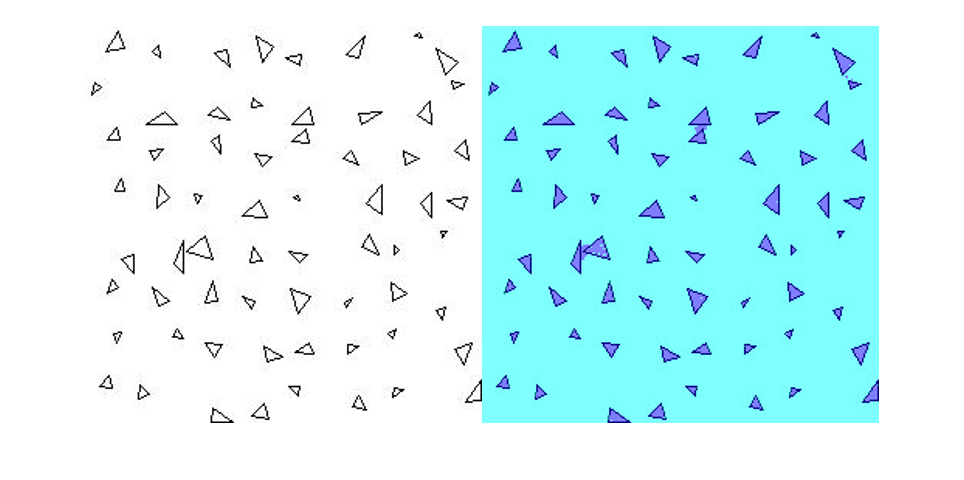Segment the test image using `semanticseg` and display the results using `labeloverlay`.

```C = semanticseg(imgTest,net); B = labeloverlay(imgTest,C); figure imshow(B)```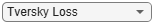References

1. Chen, Liang-Chieh, George Papandreou, Iasonas Kokkinos, Kevin Murphy, and Alan L. Yuille. "Deeplab: Semantic image segmentation with deep convolutional nets, atrous convolution, and fully connected crfs." IEEE transactions on pattern analysis and machine intelligence 40, no. 4 (2018): 834-848.

## Tips

• The `pixelLabelDatastore` `pxds` and the `imageDatastore` `imds` store files that are located in a folder in lexicographical order. For example, if you have twelve files named `'file1.jpg'`, `'file2.jpg'`, … , `'file11.jpg'`, and `'file12.jpg'`, then the files are stored in this order:

````'file1.jpg'` `'file10.jpg'` `'file11.jpg'` `'file12.jpg'` `'file2.jpg'` `'file3.jpg'` ... `'file9.jpg'````
Files that are stored in a cell array are read in the same order as they are stored.

If the order of files in `pxds` and `imds` are not the same, then you may encounter a mismatch when you read a ground truth image and corresponding label data using a `pixelLabelImageDatastore`. If this occurs, then rename the pixel label files so that they have the correct order. For example, rename `'file1.jpg'`, … , `'file9.jpg'` to `'file01.jpg'`, …, `'file09.jpg'`.

• To extract semantic segmentation data from a `groundTruth` object generated by the Video Labeler or Ground Truth Labeler, use the `pixelLabelTrainingData` function.